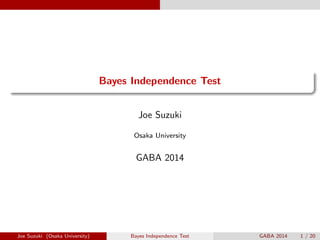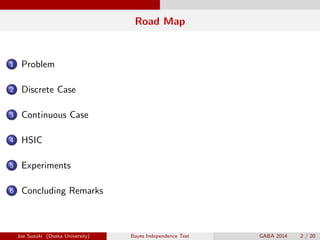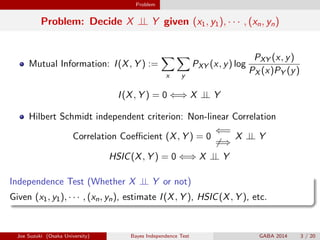Successfully reported this slideshow.

# Bayes Independence Test

1

Share×
1 of 20
1 of 20

# Bayes Independence Test

1

Share

Joe Suzuki, ``Bayes Independence Test", GABA, Yokohama, Japan, 2014

Joe Suzuki, ``Bayes Independence Test", GABA, Yokohama, Japan, 2014

## More Related Content

### Related Books

Free with a 14 day trial from Scribd

See all

### Related Audiobooks

Free with a 14 day trial from Scribd

See all

### Bayes Independence Test

1. 1. . ...... Bayes Independence Test Joe Suzuki Osaka University GABA 2014 Joe Suzuki (Osaka University) Bayes Independence Test GABA 2014 1 / 20
2. 2. Road Map ...1 Problem ...2 Discrete Case ...3 Continuous Case ...4 HSIC ...5 Experiments ...6 Concluding Remarks Joe Suzuki (Osaka University) Bayes Independence Test GABA 2014 2 / 20
3. 3. Problem Problem: Decide X ⊥⊥ Y given (x1, y1), · · · , (xn, yn) Mutual Information: I(X, Y ) := ∑ x ∑ y PXY (x, y) log PXY (x, y) PX (x)PY (y) I(X, Y ) = 0 ⇐⇒ X ⊥⊥ Y Hilbert Schmidt independent criterion: Non-linear Correlation Correlation Coeﬃcient (X, Y ) = 0 ⇐= ̸=⇒ X ⊥⊥ Y HSIC(X, Y ) = 0 ⇐⇒ X ⊥⊥ Y . Independence Test (Whether X ⊥⊥ Y or not) .. ......Given (x1, y1), · · · , (xn, yn), estimate I(X, Y ), HSIC(X, Y ), etc. Joe Suzuki (Osaka University) Bayes Independence Test GABA 2014 3 / 20
4. 4. Discrete Case Estimating MI (Maximum Likelihood) X, Y : discrete In(xn , yn ) := ∑ x ∑ y ˆPn(x, y) log ˆPn(x, y) ˆPn(x)ˆPn(y) ˆPn(x, y): relative occurency of (X, Y ) = (x, y) in (x1, y1), · · · , (xn, yn) ˆPn(x): relative occurency of X = x in x1, · · · , xn ˆPn(y): relative occurrency of Y = y in y1, · · · , yn In(x, y) → I(X, Y ) (n → ∞) even if X ⊥⊥ Y , In(xn, yn) > 0 occurs infnitely many times constructing Independent Test requires thresholds {ϵn} s.t. In(xn , yn ) < ϵn ⇐⇒ X ⊥⊥ Y cannot be extended into the case when X, Y are continuous Joe Suzuki (Osaka University) Bayes Independence Test GABA 2014 4 / 20
5. 5. Discrete Case Bayesian Estimation of MI (Proposal) . Lempel-Ziv (lzh, gzip etc.) .. ...... Compressing xn = (x1, · · · , xn) into zm = (z1, · · · , zm) ∈ {0, 1}m ...1 The compression ratio m n converges to its Entropy H(X) for any PX . ...2 ∑ 2−m ≤ 1 (Kraft’s inequality) for Qn X (xn) := 2−m, m = − log Qn X (xn) will be lenth after compression for Qn Y (yn), Qn XY (xn, yn), and prior p of X ⊥⊥ Y , Jn(xn , yn ) := 1 n log (1 − p)Qn XY (xn, yn) pQn X (xn)Qn Y (yn) Joe Suzuki (Osaka University) Bayes Independence Test GABA 2014 5 / 20
6. 6. Discrete Case MDL(minimum description length) Principle From examples, a model s.t. the total length of description of the model description of the examples given the model is minimized should be chosen (Rissanen, 1976) MDL(X ⊥⊥ Y ) := − log p − 1 n log Qn X (xn ) − 1 n log Qn Y (yn ) MDL(X ̸⊥⊥ Y ) := − log(1 − p) − 1 n log Qn XY (xn , yn ) . Consistency .. ......The MDL model coincides with the true model with. prob.1 as n → ∞. Joe Suzuki (Osaka University) Bayes Independence Test GABA 2014 6 / 20
7. 7. Discrete Case Bayesian Estimation of MI (Proposal, cont’d) Consistency of MDL implies that of Independence Test: Jn(xn , yn ) ≤ 0 ⇐⇒ MDL(X ⊥⊥ Y ) ≤ MDL(X ̸⊥⊥ Y ) for α := |X|, β := |Y | Jn(xn , yn ) ≈ In(xn .yn ) − (α − 1)(β − 1) 2n log n Jn(xn , yn ) ≤ 0 ⇐⇒ In(xn , yn ) ≤ ϵn := (α − 1)(β − 1) 2n log n Jn(xn, yn) → I(X, Y ) (n → ∞) O(n) computation p = 1 2 was assumed in Suzuki 2012. Joe Suzuki (Osaka University) Bayes Independence Test GABA 2014 7 / 20
8. 8. Discrete Case Universality: Discrete For any PX , m n = − 1 n log Qn X (xn ) → H(X) From i.i.d. and the law of large numbers, for any PX , − 1 n log Pn X (xn ) = − 1 n n∑ i=1 log PX (xi ) → E[− log PX (X)] = H(X) For any PX , 1 n log Pn X (xn) Qn X (xn) → 0 . Joe Suzuki (Osaka University) Bayes Independence Test GABA 2014 8 / 20
9. 9. Continuous Case Universality: Continuous Under regularity, there exists gn X s.t. for any fX , 1 n log f n X (xn) gn X (xn) → 0 ∫ ∞ −∞ gn (xn )dx ≤ 1 (Ryabko 2009) removing regularity even for more than one variables either discrete, continuous, or none of them (Suzuki 2013) Joe Suzuki (Osaka University) Bayes Independence Test GABA 2014 9 / 20
10. 10. Continuous Case Construcion of gn X Quantization at level k: xn = (x1, · · · , xn) → (a (k) 1 , · · · , a (k) n ) ... ... ... ... E E E Level 1 Level 2 Level k Qn 1 (a (1) 1 , · · · , a (1) n ) λ(a (1) 1 ) · · · λ(a (1) n ) Qn 2 (a (2) 1 , · · · , a (2) n ) λ(a (2) 1 ) · · · λ(a (2) n ) Qn k (a (k) 1 , · · · , a (k) n ) λ(a (k) 1 ) · · · λ(a (k) n ) wi > 0 , ∑ i wi = 1 , gn X (xn ) = ∑ i wi Qn i (a (i) 1 , · · · , a (i) n ) λ(a (i) 1 ) · · · λ(a (i) n ) Joe Suzuki (Osaka University) Bayes Independence Test GABA 2014 10 / 20
11. 11. Continuous Case Bayesian Estimation of MI: General Case . Bayesian Estimation of MI .. ...... Jn(xn , yn ) := 1 n log (1 − p)gn XY (xn, yn) pgn X (xn)gn Y (yn)   Generalization of MDL: MDL(X ⊥⊥ Y ) := − log p − 1 n log gn X (xn ) − 1 n log gn Y (yn ) MDL(X ̸⊥⊥ Y ) := − log(1 − p) − 1 n log gn XY (xn , yn ) . Consistency .. ...... The MDL model coincides with the true model with prob. 1 as n → ∞: X ⊥⊥ Y ⇐⇒ MDL(X ⊥⊥ Y ) ≤ MDL(X ̸⊥⊥ Y ) Joe Suzuki (Osaka University) Bayes Independence Test GABA 2014 11 / 20
12. 12. Continuous Case Jn(xn , yn ) → I(X, Y ) (n → ∞) Proof: since xn, yn are i.i.d., from the law of large numbers, for any fX , 1 n log f n XY (xn, yn) f n X (xn)f n Y (xn) = 1 n n∑ i=1 log f n XY (xn, yn) f n X (xn)f n Y (xn) → E[log fXY (XY ) fX (X)fY (Y ) ] = I(X, Y ) Jn(xn , yn ) − I(X, Y ) = − 1 n log f n XY (xn, yn) gn XY (xn, yn) + 1 n log f n X (xn) gn X (xn) + 1 n log f n Y (yn) gn Y (yn) + 1 n log f n XY (xn, yn) f n X (xn)f n Y (xn) − I(X, Y ) + 1 n log 1 − p p → 0 Joe Suzuki (Osaka University) Bayes Independence Test GABA 2014 12 / 20
13. 13. HSIC HSIC A nonlinear corralation coeﬃcient cov(X, Y ) Random Variable X Y Hilbert Space X Y RKHS F: Basis {fi } G: Basis {gj } kernel k : X × X → R l : Y × Y → R HSIC(PXY , F, G) = ∑ i,j cov(fi (X), gj (Y ))2 For the universal kernels, HSIC(PXY , F, G) = 0 ⇐⇒ X ⊥⊥ Y ex: the Gaussian kernel is known to be universal: k(x, y) = exp{−(x − y)2 /2} Joe Suzuki (Osaka University) Bayes Independence Test GABA 2014 13 / 20
14. 14. HSIC Limitions of HSIC . Unbiased Estimator of HSIC(PXY , F, G) .. ...... For K = (k(xi , xj )), L = (l(yi , yj )), H = (δi,j − 1 n ) HSIC(xn , yn ) = 1 (n − 1)2 tr(KHLH) HSIC(PXY , F, G) → HSIC(PXY , F, G) as n → ∞ has been proved only for weak consistncy. Computation of HSIC(xn, yn, F, G): O(n3) Computation of the asymptotic distribution of H0: is O(n3 ) w.r.t. n based on U-statistics (Bunlphone, et. al, 2014). may not give correct estimaton based on permutation test. Joe Suzuki (Osaka University) Bayes Independence Test GABA 2014 14 / 20
15. 15. Experiments Experiments ...1 E¨¨ ¨¨¨¨¨B Errr rrrrj X Y 0 1 0 1 1 2 1 2 p 1 − p I(X, Y ) = HSIC(X, Y ) = 0 ⇐⇒ p = 1 2 ⇐⇒ X ⊥⊥ Y ...2 (X, Y ) ∼ N(0, Σ), Σ = [ 1 ρ ρ 1 ] , −1 < ρ < 1 I(X, Y ) = HSIC(X, Y ) = 0 ⇐⇒ ρ = 0 ⇐⇒ X ⊥⊥ Y ...3 P(X = 0) = P(X = 1) = 1 2 , Y ∼ N(aX, 1), a ≥ 0 I(X, Y ) = HSIC(X, Y ) = 0 ⇐⇒ a = 0 ⇐⇒ X ⊥⊥ Y Joe Suzuki (Osaka University) Bayes Independence Test GABA 2014 15 / 20
16. 16. Experiments Experiment 1 The Error Probabilities for n = 100 True p Proposal HSIC →Estimated p Threshold (×10−4) 4 8 12 16 20 p = 0.5 → p ̸= 0.5 0.084 0.306 0.135 0.077 0.043 0.022 p = 0.4 → p = 0.5 0.758 0.507 0.694 0.787 0.860 0.908 p = 0.3 → p = 0.5 0.333 0.139 0.251 0.396 0.505 0.610 p = 0.2 → p = 0.5 0.048 0.018 0.035 0.083 0.135 0.201 p = 0.1 → p = 0.5 0.001 0.000 0.001 0.005 0.010 0.021 ↑ Joe Suzuki (Osaka University) Bayes Independence Test GABA 2014 16 / 20
17. 17. Experiments Experiments 2 The Error Probabilities for n = 100 ρ Proposal HSIC Threshold (×10−3) 2 4 6 8 0.0 0.095 0.338 0.036 0.006 0.00 0.2 0.628 0.298 0.676 0.884 0.97 0.4 0.168 0.008 0.088 0.300 0.512 0.6 0.008 0.000 0.000 0.002 0.006 0.8 0.000 0.000 0.000 0.000 0.000 ↑ For the Gaussian kernel and Gauss distributions, HSIC performs very well. Joe Suzuki (Osaka University) Bayes Independence Test GABA 2014 17 / 20
18. 18. Experiments HSIC shows poor performance in cases such as z        ~ \$\$\$\$\$\$\$\$\$X z\$\$\$\$\$\$\$\$\$X z& & & & & & & &&b \$\$\$\$\$\$\$\$\$X X Y 0 ϵ 1 1 − ϵ 0 ϵ 1 1 − ϵif ϵ > 0 small. HSIC(xn .yn ) = 1 (n − 1)2 ∑ i ∑ j {k(xi , xj ) − 1 n ∑ h k(xi , xh)}{l(yi , yj ) − 1 n ∑ h l(yi , yh)} k(u, v) = l(u, v) = exp{−(u − v)2 } Joe Suzuki (Osaka University) Bayes Independence Test GABA 2014 18 / 20
19. 19. Experiments Execution Time Execution (sec) n 100 500 1000 2000 Proposal 0.30 0.33 0.62 1.05 HSIC 0.50 9.51 40.28 185.53 Joe Suzuki (Osaka University) Bayes Independence Test GABA 2014 19 / 20
20. 20. Concluding Remarks Concluding Remarks . Contribution .. ......Independence Test based on MDL/Bayes Proposal HSIC Principle Bayes Detection will be maximied Strong Discrete Continuous Threshold Not Necessay Necessary Prior Necessary Not Necessary Computation O(n) O(n3) Consistency Strong Weak Future Works The Border for which either Bays/MDL or HSIC outperforms R Package Joe Suzuki (Osaka University) Bayes Independence Test GABA 2014 20 / 20